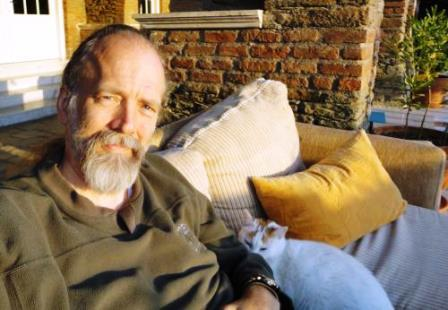The Compactness Theorem David Pierce Department of Mathematics Mimar Sinan Fine Arts University, Turkey Let us say that a logic has the compactness property if a set of sen- tences of the logic has a model whenever every finite subset of the set has a model. For present purposes, the Compactness  Theorem is that first-order logic has the compactness property.  This theorem is fundamental to model theory. However, Hodges’s comprehensive twelve-chapter 1993 volume Model Theory finds no need to state and prove the theorem until Chapter 6. It is worthwhile to think about what needs Compactness  and what does not. One consequence of the Compactness Theorem is that a set of sen- tences  with  arbitrarily   large finite  models must have  an infinite model.  A  more purely  mathematical consequence  is  the  Prime Ideal  Theorem:   every nontrivial  commutative ring  has a prime ideal. One can prove this by noting first that every maximal ideal is prime.  Moreover, every countable ring has a maximal ideal; for we can obtain a generating set of such an ideal by considering the elements of the ring one by one. In particular then, every finitely generated subring of a given ring has a maximal ideal, because every finitely generated ring is countable. By the Compactness Theorem then, the  original ring must  have an ideal that  is at least  prime, although it might not be maximal. The point here is that primeness is a “local” property, while maximality is not. It is usually understood that every nontrivial commutative ring has, not just a prime ideal, but a maximal ideal. To make it easy to prove such results, Zorn stated in 1935  the result now known by his name. However, Zorn’s Lemma relies on the Axiom of Choice. The Com- pactness Theorem is strictly  weaker than this, with  respect to ZF (Zermelo–Fraenkel set theory without Choice). For, Compactness is also a consequence  of the Prime Ideal Theorem, even the Boolean Prime Ideal Theorem; and this is strictly weaker than the Axiom of Choice (as shown by Halpern and Lévy in 1971). The Compactness  Theorem for countable  sets  of sentences  needs nothing beyond ZF. Skolem showed this implicitly  in 1922 when he established the paradox that Zermelo’s axioms for set theory must have a countable model, if they have a model at all. In 1930, Gödel proved countable Compactness explicitly, though not by that name. Mal'tsev stated the full Compactness Theorem  as the General Local Theorem  in 1941,  having proved it implicitly  in 1936;  he used it to prove  algebraic results  in the  way we proved the  Prime Ideal Theorem above. In his 1950  address to the International Congress of Mathemati- cians, Tarski gave the Compactness Theorem its current name and noted its topological meaning.  But  this meaning is not generally well expressed in today’s textbooks of model theory. The class of structures having a given signature can be given a topol- ogy, although the  closed “sets” in this  topology  are not sets, but proper  classes (except for the  empty  set):  they are the  classes of models of sets of sentences.  The space of all structures has a Kol- mogorov (T0 ) quotient  that  is  a set:  it is the  space  of complete theories of structures.  If one replaces  sentences with  their logical equivalence  classes, then the set of sentences becomes a Boolean algebra, called a Lindenbaum algebra; and the complete theories of structures become ultrafilters of the Lindenbaum algebra. By means of the Boolean Prime Ideal Theorem, the Stone space consisting  of all ultrafilters of the Lindenbaum algebra is easily shown to be com- pact.  Or one could look instead at the spectrum, consisting of the prime ideals of the corresponding Boolean ring; the spectrum of ev- ery ring is compact.  The Compactness Theorem says more: every ultrafilter of the Lindenbaum algebra is derived from the complete theory of a structure. The compactness theorem for propositional logic can be seen as a version of the theorem that the product of two-element discrete spaces (or indeed any compact Hausdorff  spaces) is compact. The Compactness Theorem  for first-order logic does not follow so readily, though it can be seen to result from a kind of reduction of first-order logic to propositional logic.  Then Lindström’s Theorem is roughly that there  is  no such reduction  for certain more expressive logics—but see that tutorial  for more. Sometimes the Compactness Theorem is derived from the Completeness Theorem: see that tutorial for more. Meanwhile, the present tutorial  is intended to fill out the foregoing sketch of the Compactness Theorem as such.Bibliography  JohnW. Dawson, Jr. The compactness of first-order logic: from Gödel to Lindström. Hist. Philos. Logic, 14(1):15–37, 1993.  Kurt Gödel. The completeness of the axioms of the functional calculus of logic. In van Heijenoort , pages 582–91. First published 1930.  J. D. Halpern and A. Lévy. The Boolean prime ideal theorem does not imply the axiom of choice. In Axiomatic Set Theory (Proc. Sympos. Pure Math., Vol. XIII, Part I, Univ. California, Los Angeles, Calif., 1967), pages 83–134. Amer. Math. Soc., Providence, R.I., 1971.  Wilfrid Hodges. Model theory, volume 42 of Encyclopedia of Mathematics and its Applications. Cambridge University Press, Cambridge, 1993.  Anatoli Ivanovic Malcev. The metamathematics of algebraic systems. Collected papers: 1936–1967. North-Holland Publishing Co., Amsterdam-London, 1971. Translated, edited, and provided with supplementary notes by Benjamin Franklin Wells, III, Studies in Logic and the Foundations of Mathematics, Vol. 66.  Thoralf Skolem. Some remarks on axiomatized set theory. In van Heijenoort , pages 290–301. First published 1923.  Alfred Tarski. Some notions and methods on the borderline of algebra and metamathematics. In Proceedings of the International Congress of Mathematicians, Cambridge, Mass., 1950, vol. 1, pages 705–720. Amer. Math. Soc., Providence, R. I., 1952.  Jean van Heijenoort, editor. From Frege to Gödel: A source book in mathematical logic, 1879–1931. Harvard University Press, Cambridge, MA, 2002.  Ernst Zermelo. Investigations in the foundations of set theory I. In van Heijenoort , pages 199–215. First published 1908.  Max Zorn. A remark on method in transfinite algebra. Bull. Amer. Math. Soc., 41(10):667–670, 1935.